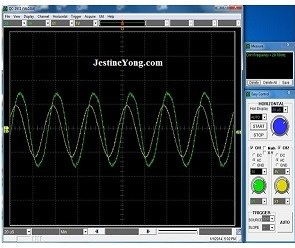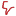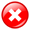Don't Miss

# An alternative way to find out the value of a small inductor

By on January 6, 2014If you need to measure the Inductance (L) of an unmarked inductor, the LCR meter is the obvious method. For some reasons (I found out much later why!) my LCR meter refused to cooperate that day. It was displaying unstable and unreliable values. When I tried with a few branded inductors (color coded or marked) the values measured were all wrong.

I wanted the job done; therefore I decided to find an alternative way. The idea was to use the resonance of a LC circuit, connecting a capacitor in parallel with the inductor to be measured. If I could find out the resonant frequency I would be able to calculate the inductance. Here is the test circuit:I used a 1 kΩ Resistor, a 0.01 µF capacitor, a simple function generator and my trusted USB oscilloscope. The function generator is connected between A and B and set for sine wave. The channel 1 of the oscilloscope (Green) is connected between A and B to measure V1, and the channel 2 of the oscilloscope (Yellow) between A and C to measure V2. Here are the settings:Now observe what happens when we slowly increase the frequency. At first V2 (Yellow) is smaller than V1 (Green). Then suddenly V2 starts to increase, to reach a maximum and then decreases. The resonant frequency is when V2 is at its maximum.Before the resonance, V2 (Yellow) is smaller than V1 (Green). Please note that V2 is also shifted to the left compared to V1.At the resonant frequency, V2 is nearly equal to V1 and there is no phase shift.After the resonance, V2 is decreasing and is now shifted to the right.

The resonant frequency, for this particular LC parallel circuit, is 36.76 KHz, as indicated by the oscilloscope.

Now we need to do some numbers crunching, not so hard though! First let’s remind a few AC circuit principles:

–       The resonance is achieved when the Inductor’s reactance (XL) is equal to the Capacitor’s reactance (XC). Hence, for this particular LC circuit, at 36.76 kHz:

Note: If you dig into theory books (or Google!) you will find out that XL = – XC but, for our purpose, we can ignore the minus sign…

–       At the resonant frequency, the AC resistance (Impedance) of the LC parallel circuit is very high. This is why V2 is nearly equal to V1 since the voltage drop into the 1K resistor is very low (because the current is very low). The circuit is purely resistive at this frequency; this is why there is no phase shift.

Note: V1 is also increasing and reaching a maximum because of the relatively high output resistance of my function generator. This will depend on the model of function generator you are using.(I know it looks a bit tough, but look at the spreadsheet simplification below!)

Bingo! The accuracy of the measurement depends on the accuracy of the capacitor. The capacitor used for this experiment is rated 5% (2A103J). We are within the range!I tried this method with various small inductors, from 100 µH until 100 mH, it worked fine. To make it easier I entered the formulas in a spreadsheet, so I just type the frequency into the corresponding cell (B3) for each measurement to get the result immediately. Here is a screen dump of the spreadsheet, where I included the formulas for each cell:For some inductors I had to choose another value for the capacitor in order to bring the resonance to the middle of one of the frequency range of the function generator. Indeed, if the resonant frequency falls between two ranges of the generator (or close to the end of a range) it is difficult to see where the maximum of V2 is. Using the spreadsheet it is just a matter of entering the new capacitor value into the corresponding cell (B6).

If it might look a bit complicated (of course the LCR meter is much easier, when it works!) it is a nice classroom exercise to illustrate and understand the resonance of a parallel circuit.You may check his other articles at:

https://www.jestineyong.com/building-your-own-rf-field-strength-meter/

and

https://www.jestineyong.com/double-insulated-smps/

Note: If you have any interesting repair article that you want to share to others please do email me HERE

Please give a support by clicking  on the social buttons below. Your feedback on the post is welcome. Please leave it in the comments-thanks.

Likes(11)Dislikes(0)This site uses Akismet to reduce spam. Learn how your comment data is processed.

Open